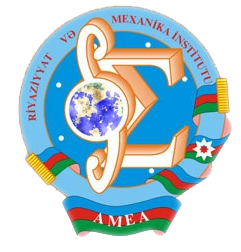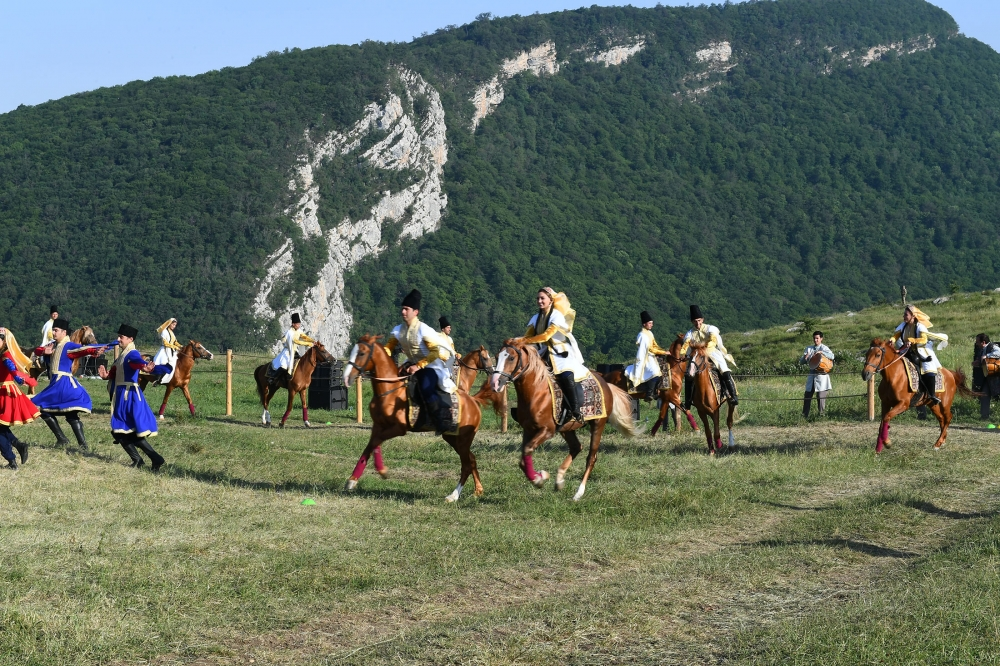Riyaziyyat və Mexanika İnstitutu

## Qarabağ xəbərləri### Şuşada Heydər Əliyev Fondunun təşkilatçılığı ilə “Musiqi irsi və Qarabağ atları Cıdır düzündə” adlı kompozisiya təqdim olunub## Transactions 2011/4/XXXI

 Year: 2011 Volume: 4 Number: XXXI MATHEMATICS Aliyev A.B., Kazimov A.A. Abstract PDF Global solvability and the behavior of solutions for Cauchy problem for systems of three semilinear hyperbolic equations with dissipation [PDF] 3 Aliyeva L.R. Abstract [PDF] Equivalent norms in mean oscillation spaces [PDF] 19 Aslanova N.M., Aslanov Kh.M. Abstract [PDF] On identity for eigenvalues of one boundary value problem with eigenvalue dependent boundary condition [PDF] 27 Aslanov G.I., Badalova K.G. Abstract [PDF] Investigation of Green function of higher order operator-differential equation on ﬁnite segment [PDF] 35 Bilalov B.T., Farahani S.M. Abstract [PDF] On perturbed bases of exponential functions with complex coeffcients [PDF] 45 Delshad Gharehgheshlaghi A.Y. Abstract [PDF] Investigation of a linear boundary value problem for a composite type two-dimensional differential equation of third order with general boundary conditions [PDF] 51 Guliyev V.S., Muradova Sh.A. Abstract [PDF] Parabolic fractional maximal operator in parabolic local Morrey-type spaces [PDF] 59 Huseynov R.V., Aliev N.A., Murtuzayeva S.M. Abstract [PDF] Inﬂuence of Karleman condition by investigating boundary value problems for Laplace equation [PDF] 73 Isayeva S.E. Abstract [PDF] The existence of an absorbing set for one mixed problem with memory operator [PDF] 85 Ismailov M.I. Abstract [PDF] On close b-bases [PDF] 95 Mamaedov F.I., Ibrahimov T.T. Abstract [PDF] A mean value theorem approach to the removable sets of parabolic equations [PDF] 103 Mehraliyev Y.T. Abstract [PDF] Investigation of the classical solution of a boundary value problem for a second order parabolic equation with non-classical boundary conditions [PDF] 121 Sabzaliev M.M. Abstract [PDF] Asymptotics of solution of a boundary value problem for a singularly perturbed quasilinear one-characteristic equation [PDF] 133 Yusufov V.Sh.. Abstract [PDF] Semigroups of local homeomorphic mappings [PDF] 143 APPLIED PROBLEMS OF MATHEMATICS AND MECHANICS Ahmedov N.K., Mamedova T.B. Abstract [PDF] Investigation of three-dimensional stress-strain state of a small thickness radially-inhomogeneous transversally-isotropic sphere [PDF] 147 Huseynova N.Sh., Niftiyev A.A. Abstract [PDF] On dependence of energy eigen-value on the ends of the interval [PDF] 159
Azərbaycanda COVID-19 ilə bağlı statistika
• Virusa yoluxan

598503

• Sağalan

567385

• Yeni yoluxan

0

• Aktiv xəstə

23114

• Ölüm halı

8004

• Test edilib

5,621,021